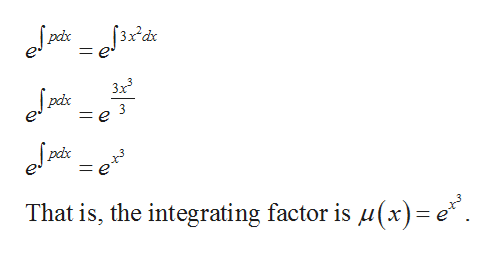# Use the method of integrating factors to solve the given initial value problem.y'+3x2y=sin(x)e-x^3, y(0)=1

Question
18 views

Use the method of integrating factors to solve the given initial value problem.

• y'+3x2y=sin(x)e-x^3, y(0)=1
check_circle

Step 1

The given equation is

Step 2

Note that, the given equation first order linear differential equation which is in the form of

Step 3

Compute the integrating f...help_outlineImage Transcriptionclosepix 3x3 pix 3 = e pc That is, the integrating factor is u(x)= e" fullscreen

### Want to see the full answer?

See Solution

#### Want to see this answer and more?

Solutions are written by subject experts who are available 24/7. Questions are typically answered within 1 hour.*

See Solution
*Response times may vary by subject and question.
Tagged in

### Math